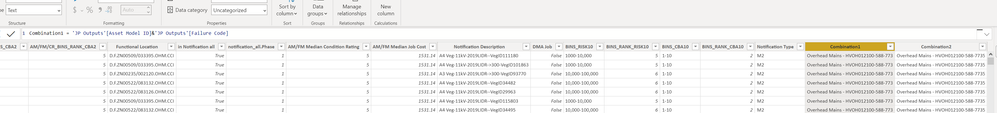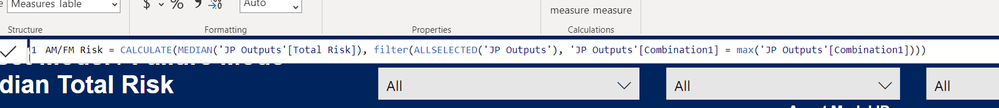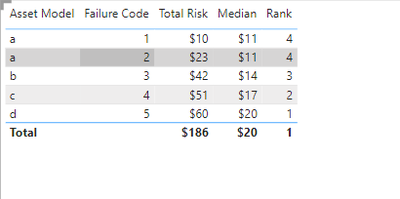cancel
Showing results for
Did you mean:Helper I

## Calculate median and median rank for a combination and change for filter

HI I have this data set

 Asset Class Asset Model Failure Code Notification Number Condition Rating Total Risk Work Centre a a 1 1 10 \$10 aa a a 2 2 9 \$11 bb a a 2 3 8 \$12 cc b b 3 4 10 \$13 aa b b 3 5 10 \$14 aa b b 3 6 10 \$15 aa c c 4 7 5 \$16 aa c c 4 8 5 \$17 aa c c 4 9 5 \$18 bb d d 5 10 6 \$19 aa d d 5 11 6 \$20 bb d d 5 12 6 \$21 aa

I want to create this table

 Asset Class Asset Model Failure Code Count of Notifications Condition Rating Median Risk Median Risk Rank a a 1 1 10 calculate this calculate this a a 2 1 9 calculate this calculate this a a 2 1 8 calculate this calculate this b b 3 3 10 calculate this calculate this c c 4 3 5 calculate this calculate this d d 5 3 6 calculate this calculate this

The thing is, I want to be able to have a slicer on the page and if I change the work centre it recalculates the median for the ones in that work centre and the rank as well.

Then, what I want to do is plot a scatter plot and have the rank on the x axis of a scatter plot and the total risk on the y axis for each notification and this changes when you filter on work centre.

1 ACCEPTED SOLUTIONSuper User
6 REPLIES 6Super UserHelper IHelper I

Hi I see your formula was calculating the median risk for Asset Class. I wanted to do it for a combination of Asset Model ID & Failure Code & Condition Rating so I made a combination of those as another column then passed that through and it seemed to work. Now when I do it in my report, the median seems to be working but the rank doesnt.I then created some measuresand then I created the rankbut for some reason the AM/FM Risk one works and I can put it in a tableBut when I try and add Rank AM/FM Risk it goes blank. Can you see what I have done wrong??Super User

Can you share sample pbix after removing sensitive data.Super User

@fingalbrad , I tried on old data, but it is workingHelper I

you are an absolute legend. That works perfectly. Thank you so much for that